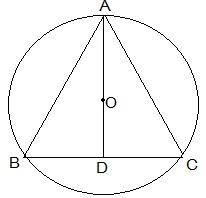# A circular park of radius 20m is situated in a colony. Three boys Ankur, Syed and David are sitting at equal distance on its boundary each having a toy telephone in his hands to talk each other. Find the length of the string of each phone.#### Solution

Given

The positions of Ankur, Syed and David are represented as A, B and C respectively.

As they are sitting at equal distances, the triangle is equilateral

AD is the median of ΔABC and it passes through the centre O.

O is the centroid of the ΔABC. OA is the radius of the triangle.

Let the side of a triangle a metres then BD = a/2 m.

On applying Pythagoras theorem in ΔABD,

20 m = 2/3 × √3a/2

a = 20√3 m

The length of the string of the toy is 20√3 m.